D3 Domain
FREE     5 minute and 25 seconds read

D3 Tutorial:

D3 Domain

D3 Domain Introduction

D3 Domain is part of the D3.js data visualizations library.

The D3 Domain function helps you go from your input data to a data representation that will fit into the space in which you want to create your chart.

We'll use D3.js version 5.9.7 for this D3 Domain example.

``````d3.version;
``````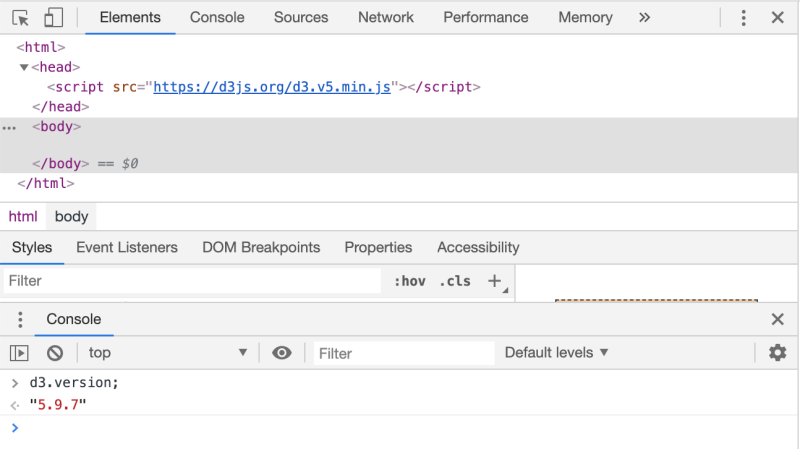D3 Domain Context Explanation

D3 Domain comes into play when using the D3 Scale.

D3 Domain is how you tell D3 what data is going to be fed into the D3 Scale.

Let's look at three D3 Domain examples:

D3 Domain Example One

D3 Domain can be used to allow you to make your small data readable.

Let's say your input data is very small as in 0.000100, 0.0001001, 0.0001002, 0.0001003 all the way through 0.0002000.

To represent this on a web page D3 chart, you need to be able to somehow translate those very small numbers into pixels that are visible on your web page.

Whether you create your dashboard with SVG or Canvas or HTML elements, you need a way to make your small numbers bigger.

You do that with the D3 Scale.

Let's define a D3 scale and tell D3 that our input data covers the values from 0.000100 to 0.000200

``````var d3_domain_example_scale = d3.scaleLinear()
.domain([0.000100, 0.000200])
.range( [0,        100     ]);
``````

Notice that the D3 Domain function is where you pass an array with how you want to map your initial data values (0.000100 to 0.000200) to your output values.

The left most value in the domain array, 0.000100, will translate to 0 and the right most value in the domain array, 0.000200, will translate to 100.

Now that we have the D3 Domain set and the d3 scale defined, let's pass in values into the d3 domain example scale to see what we get.

First, we pass in the left most data value -> 0.000100

``````d3_domain_example_scale(0.000100)
``````

And we can see the D3 Scale function returns the value 0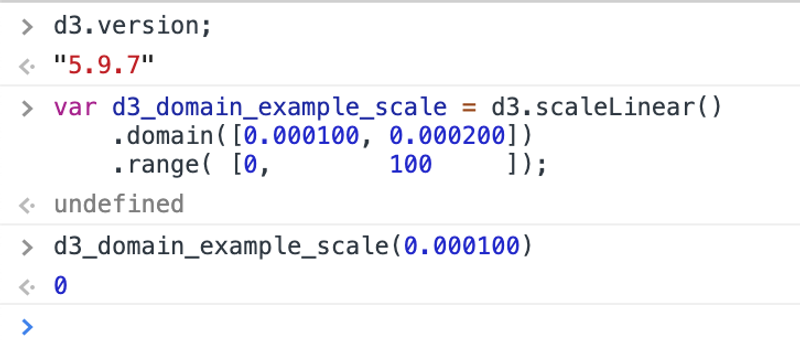Next, we pass in the right most data value -> 0.000200

``````d3_domain_example_scale(0.000200)
``````

And we can see the D3 Scale function returns the value 100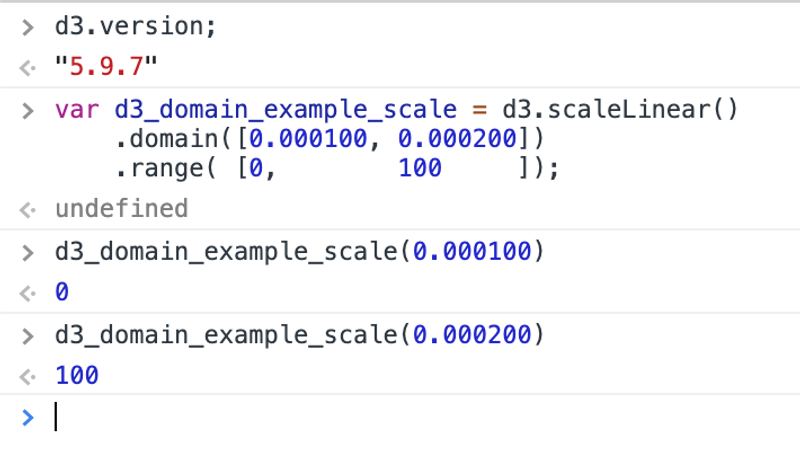Last, we pass in a value in the middle to see if the extrapolation works -> 0.000150

``````d3_domain_example_scale(0.000150)
``````

And we can see the D3 Scale function returns the value 50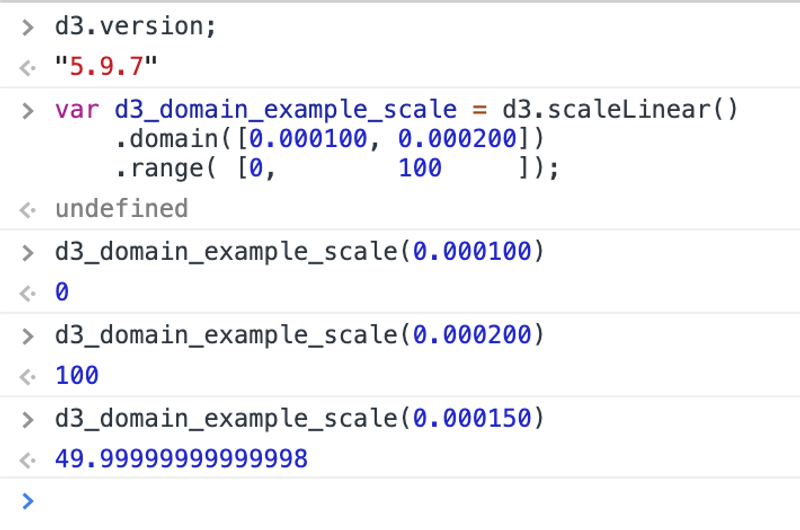Perfect - you were able to use D3 Domain to go from small data to a bigger data representation for your chart.

D3 Domain Example Two

D3 Domain can be used to allow you to make your large data readable.

Let's say your input data is large as in 1000, 1001, 1002, 1003 all the way through 2000.

To represent this on a web page D3 chart, you need to be able to somehow translate those large numbers into pixels that are visible on your web page.

Whether you create your dashboard with SVG or Canvas or HTML elements, you need a way to make your large numbers smaller.

You do that with the D3 Scale.

Let's define a D3 scale and tell D3 that our input data covers the values from 1000 to 2000

``````var d3_domain_example_scale = d3.scaleLinear()
.domain([1000, 2000])
.range( [   0,  100]);
``````

Notice that the D3 Domain function is where you pass an array with how you want to map your initial data values (1000 to 2000) to your output values.

The left most value in the domain array, 1000, will translate to 0 and the right most value in the domain array, 2000, will translate to 100.

Now that we have the D3 Domain set and the d3 scale defined, let's pass in values into the d3 domain example scale to see what we get.

First, we pass in the left most data value -> 1000

``````d3_domain_example_scale(1000)
``````

And we can see the D3 Scale function returns the value 0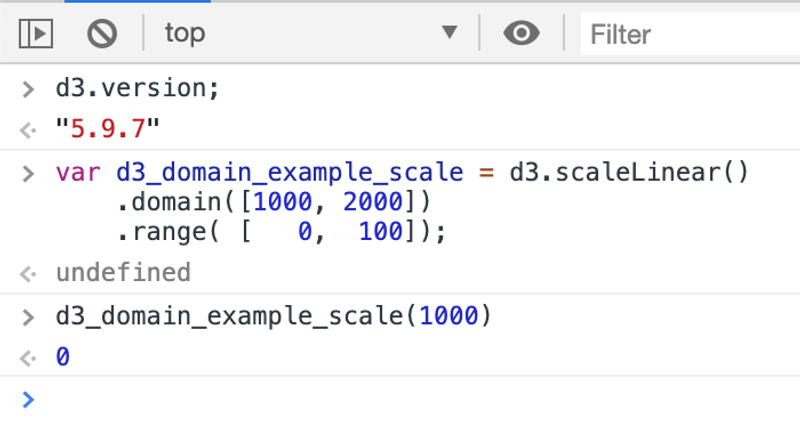Next, we pass in the right most data value -> 2000

``````d3_domain_example_scale(2000)
``````

And we can see the D3 Scale function returns the value 100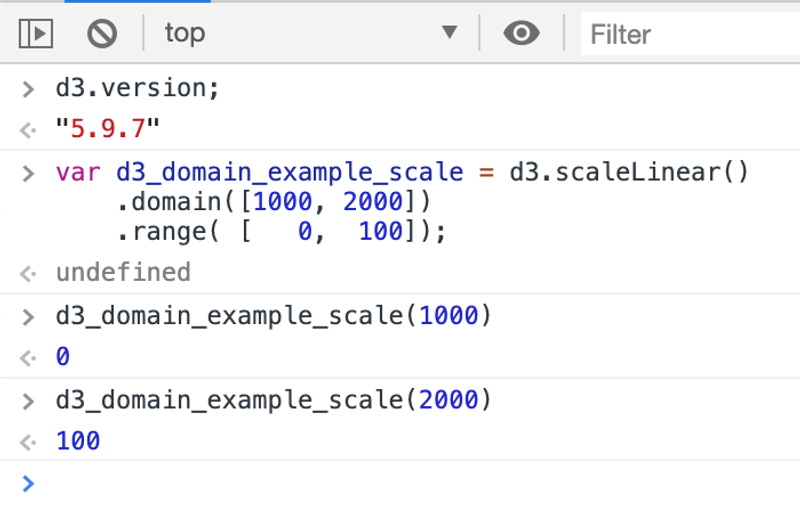Last, we pass in a value in the middle to see if the extrapolation works -> 1500

``````d3_domain_example_scale(1500)
``````

And we can see the D3 Scale function returns the value 50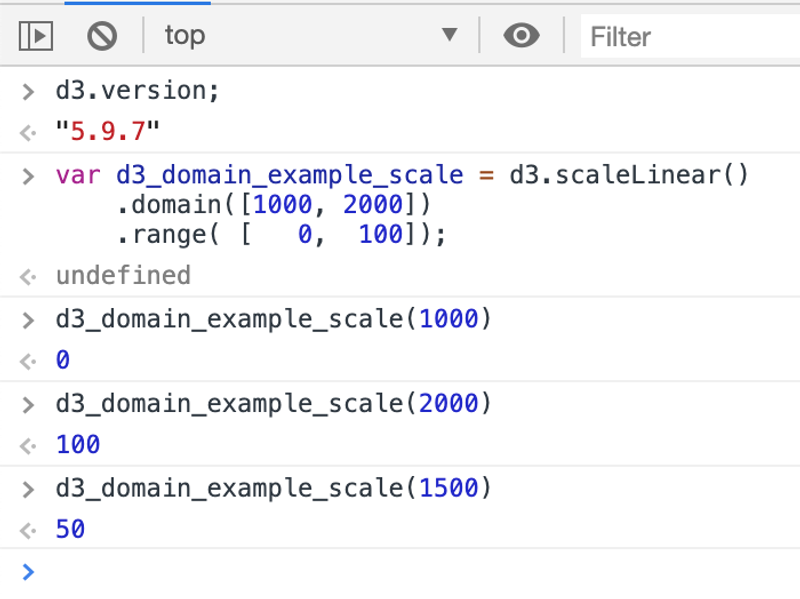Perfect - you were able to use D3 Domain to go from large to a smaller data representation for your chart.

D3 Domain Example Three

D3 Domain can be used to allow you to invert your data.

That is, if you want your data to decrease from left to right, rather than increase as it so often does in a cartesian coordinate system.

Let's say your input data goes from 2000 to 0.

And you want your 2000 to be the origin point and the 0 point to be at the right most side of the x-axis or the top most point of the y-axis.

To represent this on a web page D3 chart, you need to be able to somehow invert your data to fit into pixels that are visible on your web page.

Whether you create your dashboard with SVG or Canvas or HTML elements, you need a way to make your inverted so that it'll make sense when the data is visualized.

You do that with the D3 Scale.

Let's define a D3 scale and tell D3 that our input data covers the values from 2000 to 0.

``````var d3_domain_example_scale = d3.scaleLinear()
.domain([2000,   0])
.range( [   0, 100]);
``````

Notice that the D3 Domain function is where you pass an array with how you want to map your initial data values (2000 to 0) to your output values.

The left most value in the domain array, 2000, will translate to 0 and the right most value in the domain array, 0, will translate to 100.

Now that we have the D3 Domain set and the d3 scale defined, let's pass in values into the d3 domain example scale to see what we get.

First, we pass in the left most data value -> 2000

``````d3_domain_example_scale(2000)
``````

And we can see the D3 Scale function returns the value 0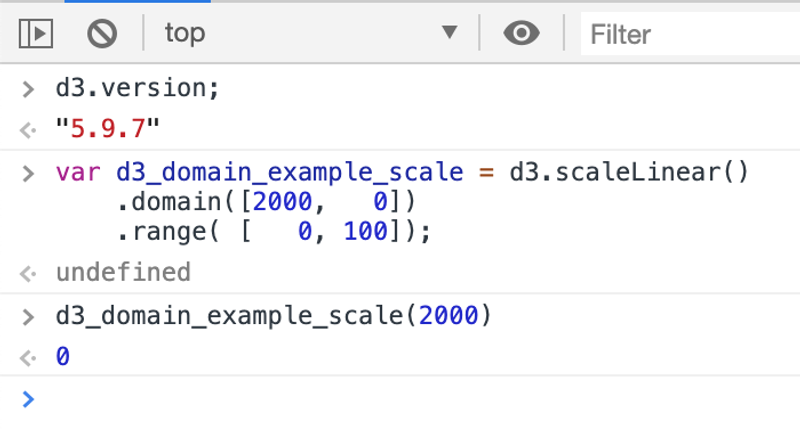Next, we pass in the right most data value -> 0

``````d3_domain_example_scale(0)
``````

And we can see the D3 Scale function returns the value 100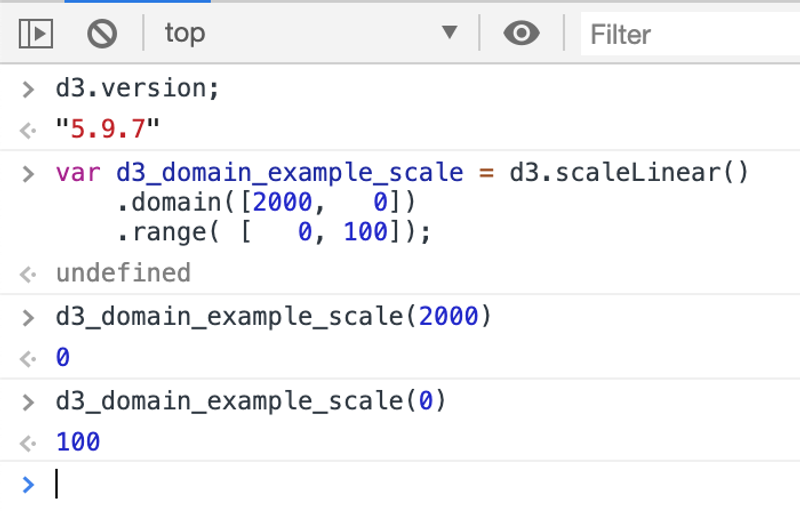Last, we pass in a value in the middle to see if the extrapolation works -> 1000

``````d3_domain_example_scale(1000)
``````

And we can see the D3 Scale function returns the value 50Perfect - you were able to use D3 Domain to go from large to a smaller data representation for your chart.

Next steps with D3 Domain

Now that you've seen how the D3 Domain function helps you go from your input data to a data representation that will fit into the space in which you want to create your chart, you'll want to try it own your own.

If you have any questions or want clarification on any of the above, please email me at sebastian@dashingd3js.com.

<< Back To D3 Screencast and Written Tutorials Index